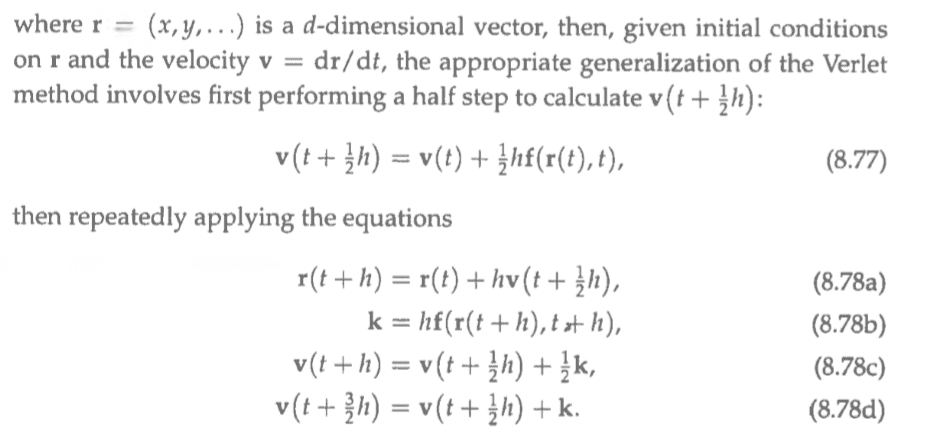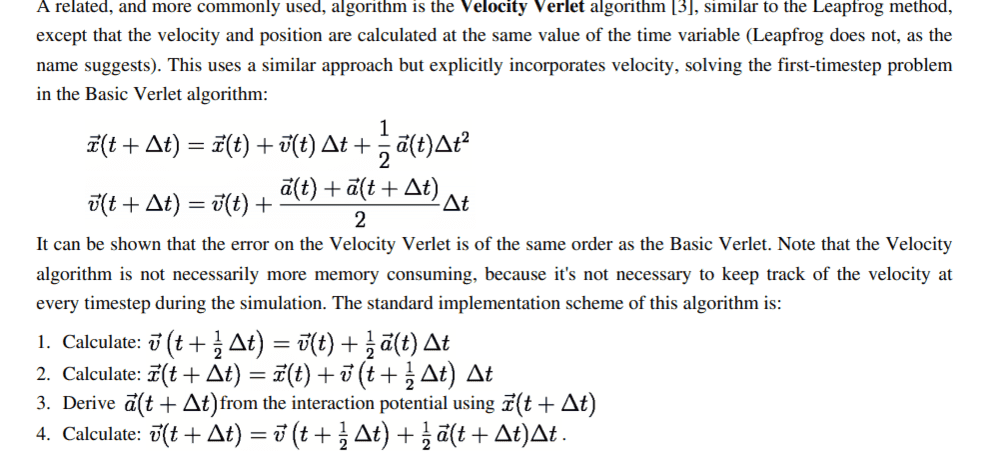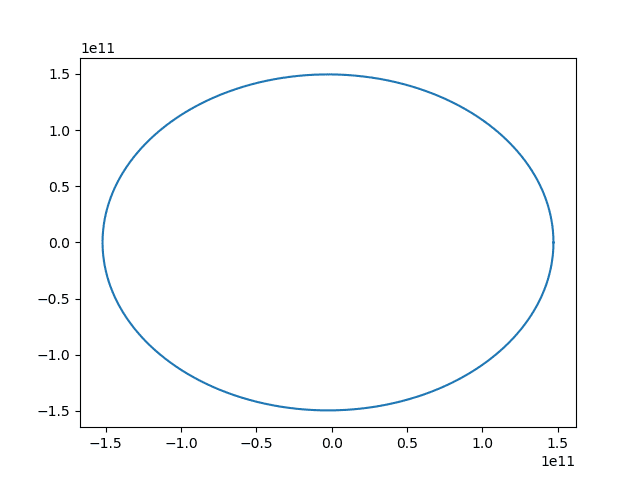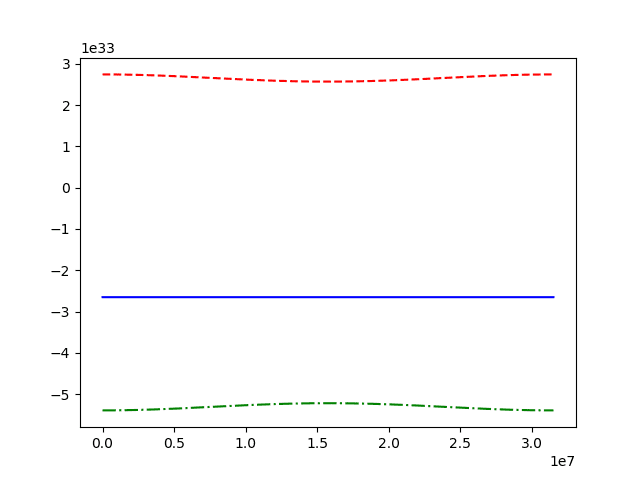# Verlet Algorithm

Gold Member
I am trying to understand the verlet algorithm but I am kind of stuck.I guess first we are findind the ##v(t + 1/2h)## then we are leaving it there and starting a loop for 8.78 ?

Also I did not understand the meaning of the equation 8.78 ? We are never using ##v(t + 3h/2)## ? Or in the loop ##v(t + 3h/2)## becomes ##v(t + 1/2h)##

Mark44
Mentor
I am trying to understand the verlet algorithm but I am kind of stuck.

View attachment 250751

I guess first we are findind the ##v(t + 1/2h)## then we are leaving it there and starting a loop for 8.78 ?
Yes, you calculate v(t + h/2) using the formula in 8.77.

No, there is no step 8.78, but there are steps 8.78a, 8.78b, and so on. And these steps aren't a loop -- you do them in succession. IOW, first you calculate r(t + h) as in 8.78a, then you calculate the vector k as in 8.78b, then v(t + h) and finally v(t + 3h/2).

Presumably there is some description of f(r(t), t) that isn't shown on the attached image.
Also part of the formula for step 8.78b is confusing in the 2nd argument to f. It looks like t + h, but maybe there is a smudge on the paper?
Arman777 said:
Also I did not understand the meaning of the equation 8.78 ? We are never using ##v(t + 3h/2)## ? Or in the loop ##v(t + 3h/2)## becomes ##v(t + 1/2h)##
It's not clear to me, either. It would be a good idea to look up Verlet algorithm to see if you can find a clearer description.

Gold Member
Yes, you calculate v(t + h/2) using the formula in 8.77.

No, there is no step 8.78, but there are steps 8.78a, 8.78b, and so on. And these steps aren't a loop -- you do them in succession. IOW, first you calculate r(t + h) as in 8.78a, then you calculate the vector k as in 8.78b, then v(t + h) and finally v(t + 3h/2).

Presumably there is some description of f(r(t), t) that isn't shown on the attached image.
Also part of the formula for step 8.78b is confusing in the 2nd argument to f. It looks like t + h, but maybe there is a smudge on the paper?
It's not clear to me, either. It would be a good idea to look up Verlet algorithm to see if you can find a clearer description.
I searched online but they are using acceleration... I guess Verlet algorithm is also not much accurate... I did not understand the idea .. I was trying to solve a "one" body problem by using RK method but it seemed dead end so I was trying to do it with verlet but I couldnt understand the steps.In Newtonian Gravity If I have $$d^2r/dt^2 = -GMr/r^3$$ so then in the above picture ##a(t)=-GMr/r^3## ?

I actually wanted to use Bulirsch-Stoer method but I am not sure how can I manage that without doing Verlet properly

Last edited:
Mark44
Mentor
In Newtonian Gravity If I have $$d^2r/dt^2 = -GMr/r^3$$ so then in the above picture ##a(t)=-GMr/r^3## ?
My copy of Halliday & Resnick shows one form of Newton's law of universal gravitation as
$$F = G\frac{m_1m_2}{r^2}$$
Your equation has just one mass in it -- M. For gravitational forces, you need to have two masses at least, with each attracting the other with forces that are equal in magnitude but opposite in direction.

Gold Member
My copy of Halliday & Resnick shows one form of Newton's law of universal gravitation as
$$F = G\frac{m_1m_2}{r^2}$$
Your equation has just one mass in it -- M. For gravitational forces, you need to have two masses at least, with each attracting the other with forces that are equal in magnitude but opposite in direction.
$$F = m \frac{d^2\vec{r}} {dt^2} = -\frac{MmG\vec{r}} {r^3}$$
so
$$\frac{d^2\vec{r}} {dt^2} = -\frac{MG\vec{r}} {r^3}$$

Gold Member
I guess I solved it ( The question claims that we should find a non circular orbit, but near elliptical one ). Here is the graph of the orbitNow I ll try to calculate the total energy of the system if it matches with the real values great!

Mark44
Mentor
Gotcha.
so then in the above picture ##a(t)=-GMr/r^3## ?
Looks fine to me. Keep in mind that r is a function of t. Also, in post #6, you're distinguishing between r in the numerator (a vector) and r in the denominator, the magnitude of the vector.

•Arman777
Gold Member
Gotcha.
Looks fine to me. Keep in mind that r is a function of t. Also, in post #6, you're distinguishing between r in the numerator (a vector) and r in the denominator, the magnitude of the vector.
Yes I was careful about that"-." lines are potential energy
"--" lines are kinetic energy and other is total energy.

My findings
Potential energy: -5.30517849127762e+33 Kinetic Energy: 2.6525789562766106e+33 Total energy: -2.6525995467214404e+33

And these values from a site
 -5.29201e+33 2.66762e+33 -2.62439e+33
I guess they are somewhat matching. Maybe I can increase the accuracy by decreasing the time step (?)

Now I took the average of the energies, more close to the actual values. (Before this I only took the last values in the data array, :p what a mistake)

Last edited:
Mark44
Mentor
Maybe I can increase the accuracy by decreasing the time step (?)
Yes, up to a point. Beyond some level, you'll be getting errors in the calculations because computers don't do perfect floating point calculations.

•Arman777
Gold Member
Yes, up to a point. Beyond some level, you'll be getting errors in the calculations because computers don't do perfect floating point calculations.
Indeed. Also the method is not so perfect. Thanks for the help

pbuk